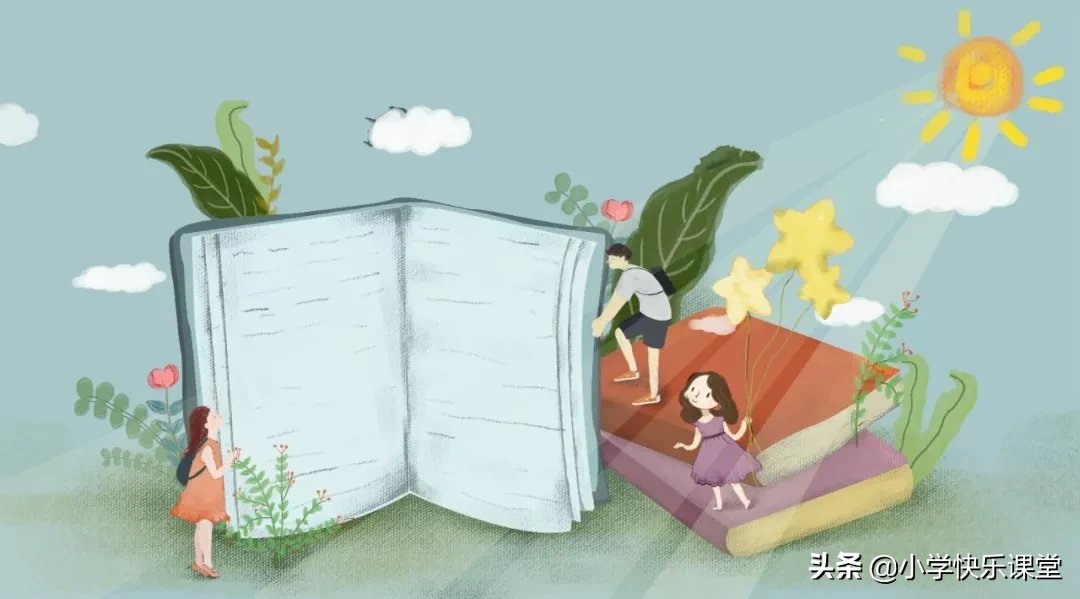七库美图  2022-07-22 15:20 七库美图 隐藏边栏 |   抢沙发  86本：

体(体操) 笨(笨重) 苯(甲苯)

皮：

波(波浪) 被(被子) 披(披风)

玻(玻璃) 坡(山坡) 跛(跛脚)

工：

扛(扛活) 杠(单杠) 江(江水)

红(红色) 贡(贡献) 虹(彩虹)

利：

梨(梨头) 俐(伶俐) 犁(犁牛)

莉(茉莉) 痢(痢疾)

肖：

宵(夜宵) 消(消息) 削(削面)

霄(云霄) 销(开销) 哨(放哨)

稍(稍微) 梢(树梢) 悄(悄悄)

峭(陡峭) 逍(逍遥)

冈：

刚(刚才) 岗(下岗) 钢(钢笔)

圭：

桂(桂花) 挂(挂面) 洼(山洼)

佳(佳节) 鲑(鲑鱼) 娃(娃娃)

令：

玲(玲珑) 怜(可怜) 拎(拎包)

伶(伶俐) 领(领巾) 邻(邻居)

零(零分) 羚(羚羊) 冷(寒冷)

岭(山岭) 铃(铃声) 龄(年龄)

者：

著(著名) 煮(煮饭) 都(首都)

诸(诸葛) 猪(猪肉)

中：

钟(时钟) 肿(红肿) 忠(忠心)

种(种树) 仲(仲秋) 冲(冲洗)

虫：

虽(虽然) 独(独立) 茧(茧子)

浊(浑浊) 触(触电) 烛(烛光)

且：

姐(姐姐) 组(组长) 祖(祖国)

县(县长) 租(房租) 阻(阻力)

彐：

扫(扫地) 雪(雪地) 妇(妇女)

灵(灵光) 当(当心)

京：

凉(凉快) 晾(晾晒) 谅(原谅)

惊(吃惊) 景(风景) 鲸(鲸鱼)

亥：

孩(孩子) 核(核心) 咳(咳嗽)

该(应该) 刻(刻苦)

兑：

说(说话) 脱(脱离) 悦(喜悦)

阅(阅读) 蜕(蜕变)

各：

客(客人) 格(格子) 阁(阁楼)

胳(胳膊) 洛(洛阳) 骆(骆驼)

路(马路) 咯(咯吱)

平：

坪(草坪) 苹(苹果) 评(评论)

怦(怦然心动) 抨(抨击)

瓜：

狐(狐狸) 孤(孤独) 弧(圆弧)

票：

漂(漂浮) 飘(飘浮) 瓢(瓢虫)

分：

芬(芬芳) 纷(纷纷) 粉(面粉)

吩(吩咐) 氛(气氛) 份(份额)

忿(忿忿) 扮(打扮) 盆(花盆)

口：

如(如果) 问(问题) 扣(扣子)

吕(姓吕) 回(回家) 员(队员)

昔：

错(错误) 惜(可惜) 借(借书)

猎(猎人) 蜡(蜡烛) 鹊(喜鹊)

占：

点(点心) 战(战友) 站(站立)

沾(沾光) 贴(贴心) 帖(帖子)

隹：

难(难过) 准(准备) 淮(淮海)

维(维护) 椎(圆椎) 谁(谁人)

堆(堆放) 推(推门) 唯(唯心)

佥：

验(实验) 检(检查) 脸(笑脸)

捡(捡拾) 俭(俭朴) 签(签名)

欠：

吹(吹风) 欢(喜欢) 炊(炊烟)

次(一次) 饮(饮料)

巴：

把(把握) 爸(爸爸) 吧(好吧)

芭(芭蕾) 笆(篱笆) 疤(伤疤)

亡：

忘(忘记) 忙(帮忙) 芒(芒果)

盲(盲人) 妄(妄想)

主：

住(住房) 往(往常) 柱(圆柱)

注(注意) 蛀(蛀牙) 拄(拄拐杖)

包：

跑(跑步) 炮(大炮) 泡(泡饭)

抱(拥抱) 饱(温饱) 苞(花苞)

胞(同胞) 袍(旗袍) 刨(刨花)

十：

叶(树叶) 什(什么) 计(计划)

艮：

跟(跟头) 根(树根) 很(很好)

恨(仇恨) 痕(伤痕) 退(退步)

垦(开垦) 眼(眼睛) 银(银行)

狠(狠心) 限(有限) 恳(诚恳)

古：

苦(辛苦) 枯(枯树) 姑(姑娘)

故(故事) 估(估计) 固(固定)